Worksheets and No Prep Teaching Resources Math Worksheets Number Line Fractions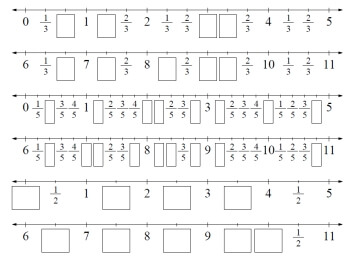Fraction Number Lines Printables, Worksheets, and Lessons

Printable Fraction Number Lines

Halves Only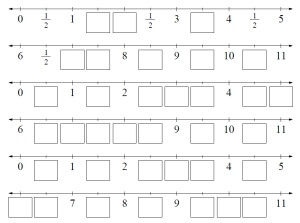One-half fractions:  Printable fraction math number Line from 0 to 11 - No blanks on first number line One-half fractions:  Printable fraction math number Line from 0 to 11
Thirds Only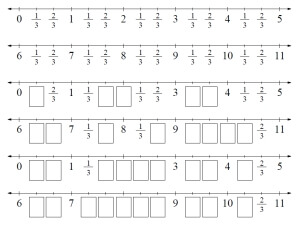Thirds only:  Printable fraction math number Line from 0 to 11 - No blanks on first number line Thirds only:  Printable fraction math number Line from 0 to 11
Fourths Only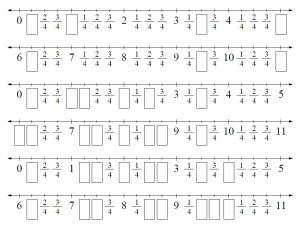Fourths only:  Printable fraction math number Line from 0 to 11 - No blanks on first number line Fourths only:  Printable fraction math number Line from 0 to 11
Fifths Only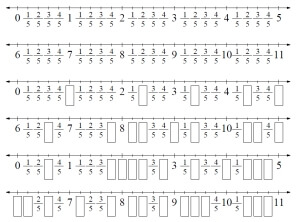Fifths only:  Printable fraction math number Line from 0 to 11 - No blanks on first number line Fifths only:  Printable fraction math number Line from 0 to 11
Mix of Halves and Thirds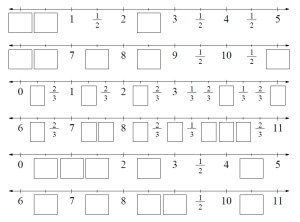Mix of Halves and Thirds:  Printable fraction math number Line from 0 to 11
Mix of Halves, Thirds, Fourths, and Fifths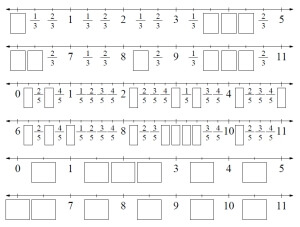Mix of Halves, Thirds, Fourths, and Fifths:  Printable fraction math number Line from 0 to 11

Have a suggestion or would like to leave feedback?